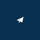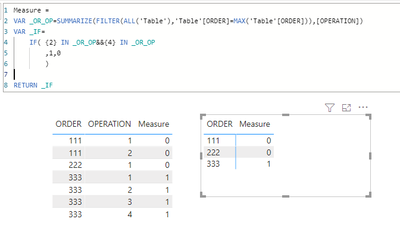cancel
Showing results for
Did you mean:Helper III

## Filter with specific definition

Hi all,

I would like to ask you for help with DAX formula for following situation:

I have this simple table with 2 columns where is order number and operation number:

 ORDER OPERATION 111 1 111 2 222 1 333 1 333 2 333 3 333 4

And I need to have the list of all orders with note if the specific order contains operation 2 and 4. So expected outcome will looks like this:

 ORDER MEASURE 111 0 222 0 333 1

0 means that order doesn´t contain operation 2 and 4 and 1 means that order contains operation 2 and 4.

Any idea how to do it?

Thanks.

P.

1 ACCEPTED SOLUTIONCommunity Support

Hi, @Peter_2020

Try to create a measure like this:

``````Measure =
VAR _OR_OP=SUMMARIZE(FILTER(ALL('Table'),'Table'[ORDER]=MAX('Table'[ORDER])),[OPERATION])
VAR _IF=
IF( {2} IN _OR_OP&&{4} IN _OR_OP
,1,0
)

RETURN _IF``````

Result:Please refer to the attachment below for details. Hope this helps.

Best Regards,
Community Support Team _ Zeon Zheng

If this post helps, then please consider Accept it as the solution to help the other members find it more quickly.

4 REPLIES 4Community Support

Hi, @Peter_2020

Try to create a measure like this:

``````Measure =
VAR _OR_OP=SUMMARIZE(FILTER(ALL('Table'),'Table'[ORDER]=MAX('Table'[ORDER])),[OPERATION])
VAR _IF=
IF( {2} IN _OR_OP&&{4} IN _OR_OP
,1,0
)

RETURN _IF``````

Result:Please refer to the attachment below for details. Hope this helps.

Best Regards,
Community Support Team _ Zeon Zheng

If this post helps, then please consider Accept it as the solution to help the other members find it more quickly.Helper III

@v-angzheng-msft Thanks a lot! it works!Super User

@Peter_2020 , Measure like

Countx(Summarize(Table, Table[Opearation], "_1",calculate(distinctcount(Table[Operation]) , filter(Table, Table[Operation] in {2,4}  ))) , if( [_1]=2,1,0) )Helper III

@amitchandak Thanks for the tip but the result is for all orders the same - value 1....